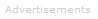# Definition of Mole

What is a Mole?

A mole corresponds to the mass of a substance that contains 6.023 x 1023 particles of the substance.

The mole is the SI unit for the amount of a substance. Its symbol is mol.

By definition: 1 mol of carbon-12 has a mass of 12 grams and contains 6.022140857 x 1023 of carbon atoms (to 10 significant figures).

Examples

1. Equations

When we write chemical equations, the quantities are assumed to be in moles. For example, in the following reaction one mole of bromine gains two moles of electrons to form two moles of bromide ions:

Br2(aq) + 2e → 2Br-

In the following example, the reactants are five moles of Fe2+, one mole of MnO4-, and 8 moles of hydrogen ions.

5Fe2+(aq) + MnO4-(aq) + 8H+(aq) → 5Fe3+(aq)) + Mn2+(aq) + 4H2O

2. Atomic and Molecular Masses

1 mol of a substance is the quantity identical to the substance's atomic or molecular mass (atomic or molecular weight). Hence:

• The atomic mass of hydrogen is 1.0079, therefore 1 mol of hydrogen atoms have a mass of 1.0079 grams.
• The atomic mass of chlorine is 35.453, therefore 1 mol of chlorine atoms have a mass of 35.453 grams.
• The molecular mass of water is 18.01528, therefore 1 mol of water molecules have a mass of 18.01528 grams.Search the Dictionary for More Terms